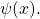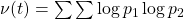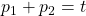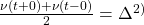# Volume 2, 1996, Number 1

Volume 2 ▶ Number 1 ▷ Number 2Number 3Number 4

On certain inequalities involving Dedekind’s arithmetical function
Original research paper. Pages 1–4
József Sándor
Full paper (PDF, 148 Kb)

Remark on φ and ψ functions
Original research paper. Pages 5–6
Krassimir T. Atanassov
Full paper (PDF, 93 Kb)

Two asymptotic formulas related to bi-unitary divisors
Original research paper. Pages 7–14
Antal Bege
Full paper (PDF, 189 Kb)

More explanations about Baica’s proof of Fermat’s last theorem
Original research paper. Pages 15–19
Malvina Baica
Full paper (PDF, 219 Kb) | Abstract

In this paper the author will answer some questions raised at some various professional conferences and meetings when she presented her proof  of Fermat’s Last Theorem.

The Euclidean character of the Fermat’s last theorem
Original research paper. Pages 20–23
Malvina Baica
Full paper (PDF, 225 Kb) | Abstract

In this paper the author is expressing her genuine concern about the proof of Fermat’s Last Theorem in the Geometry of the Elliptic Curves or Elliptic Variety which may not be equivalent to the result in the Euclidean Geometry or Euclidean Variety, where Fermat’s Last Theorem was initially originated, about three hundred fifty years ago.

On two arithmetic sets
Original research paper. Pages 24–27
Full paper (PDF, 160 Kb)

Note on some classical arithmetic functions
Original research paper. Pages 28–32
Full paper (PDF, 214 Kb)

The anatomy of even exponent Pythagorean triples
Original research paper. Pages 33–52
J. V. Leyendekkers, J. M. Rybak and A. G. Shannon
Full paper (PDF, 579 Kb) | Abstract

This paper uses a novel approach to analyse the Diophantine equation d2n = e2n + f2n in a number of ways for even and odd n, and with the use of the equivalence classes of the modular ring 6, the characteristics of primitive Pythagorean triples and right-most digit patterns.

The Goldbach problem (II)
Original research paper. Pages 53–68
Aldo Peretti
Full paper (PDF, 596 Kb) | Abstract

It is studied in first place the function, where the sum is extended for all prime numbers pi such that. Are proed formulas (14) and(26), which express its value in terms of Chebyshev’s functionIn this way is obtained formula (38), that gives the asymptotic value of s(t) with a new ”singular” series which runs through the zeros of the Zeta function, but that at present can not be evaluated in a sufficiently accurate form. In second place, for the function(with), already considered by Hardy-Littlewood in ”Partitio Numerorum III” (P.N.III), is proved the exact formulawheresecond difference;andare the direct and inverse Laplace transforms;The circle method applied in P.N.III is equivalent to determinethrough the complex inversion formula along a Bromwich contour. But it is evident that is much preferable to employ tables of direct and inverse transforms because the functions in­volved are elementary; because is obtained an exact expression for the remainder, and because all the calculus is by far more simple. One arrive thus to the unconditional formula (130), which very closely resembles the famous conjecture A of P.N.III.

Volume 2 ▶ Number 1 ▷ Number 2Number 3Number 4